Author: Oscar Cronquist Article last updated on October 05, 2020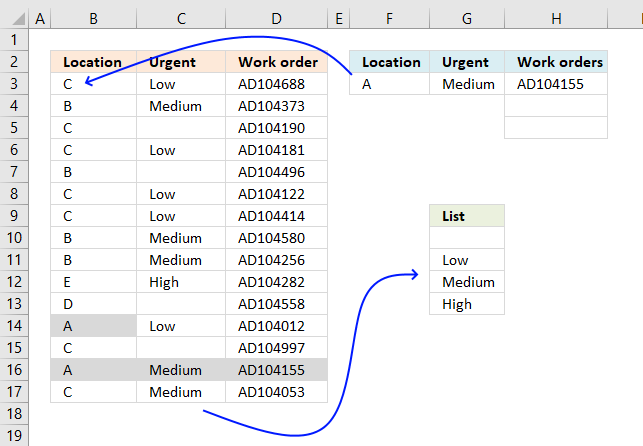This article demonstrates a formula that extracts items based on two conditions. The first condition (Location) is used to find a match in column B, the second condition is the adjacent value and its position in a list. The list (cell range G10:G13) looks like this:

• (blank)
• Low
• Medium
• High

If there are two matches (see image above, search value A matches two values) and the second condition is Low and Medium then the adjacent value to "Medium" is returned because it is further down in the list than "Low".

The formula returns values from cell range D3:D17 based on the position of the adjacent values in the above list. The image above shows that the condition in cell F3 matches the values in B14 and B16, however, the adjacent values in cell C14 and C16 determine which value to return. Cell C16 has a lower position in the list than cell C14. Value in cell D16 is returned.

This animated picture below shows you the most urgent work orders for a location. Type a location in cell F3 and the formula in cell G3 extracts the most urgent value for that particular location. The values in column C are 0 (blank), Low, Medium and High.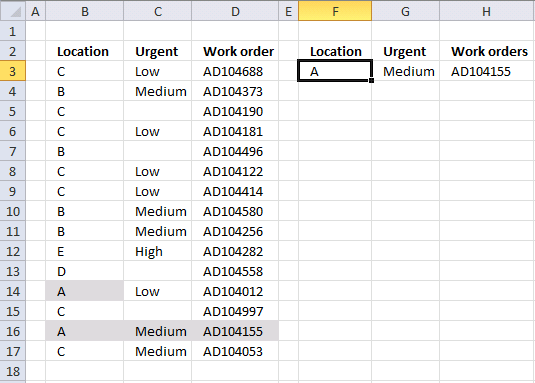I have added conditional formatting to the table so you can quickly verify that the formulas in cell G3 and H3 are correct.

Formula in cell G3:

=INDEX({""; "Low"; "Medium"; "High"}, MAX(IF(F3=B3:B17, MATCH(\$C\$3:\$C\$17, {0; "Low"; "Medium"; "High"}, 0), "")))

Formula in cell H3:

=IFERROR(INDEX(\$D\$3:\$D\$17, SMALL(IF((\$F\$3=\$B\$3:\$B\$17)*(\$G\$3=\$C\$3:\$C\$17), MATCH(ROW(\$B\$3:\$B\$17), ROW(\$B\$3:\$B\$17)), ""),ROW(A1))), "")

These formulas are array formulas if you own an Excel version previous to Excel 365 subscription.

#### How to enter an array formula

These steps are not required if you own an Excel 365 subscription.

1. Copy above formula. Shortcut keys CTRL + c.
2. Double-press with left mouse button on the destination cell.
3. Paste formula to cell. Shortcut keys CTRL + v.
4. Press and hold CTRL and SHIFT keys simultaneously.
5. Press Enter once.
6. Release all keys.

The formula bar changes, it now begins and ends with a curly bracket.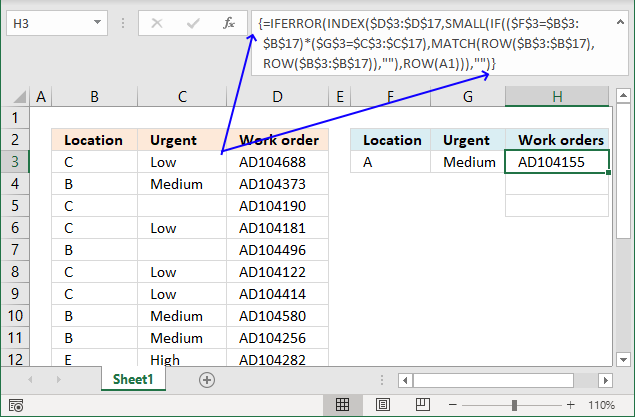This tells you that the formula is an array formula.

Note, you need to repeat the steps if you edit the formula. This is very important to remember, the formula breaks if you don't. If you begin to edit the formula and change your mind press Esc key to go back, this keeps the formula entered as an array formula and nothing is changed.

### Explaining the array formula in cell G3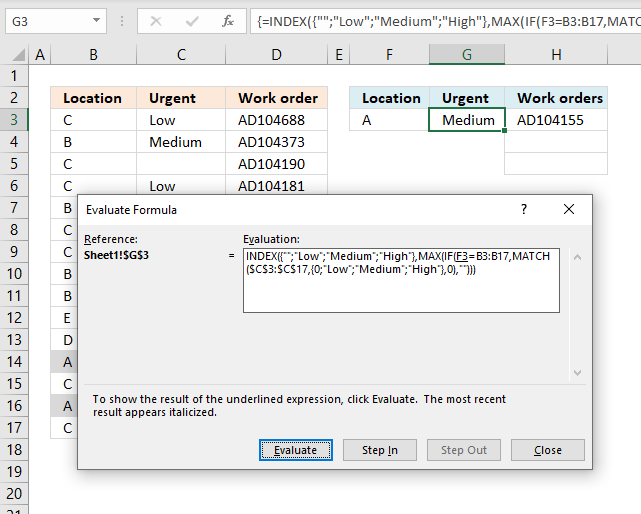It is much easier to understand and troubleshoot a formula if you use the "Evaluate Formula" tool. Select the cell containing a formula you want to examine.

Go to tab "Formulas" on the ribbon. Press with left mouse button on the "Evaluate Formula" button, a dialog box appears, see image above.

Press with left mouse button on the "Evaluate" button located on the dialog box to go through the calculations, step by step, in greater detail. The underlined expression is what is going to be evaluated next and the most recent evaluation is italicized.

#### Step 1 - Calculate the relative positions of values in column C with these values {0; "Low"; "Medium"; "High"}

The MATCH function returns the relative position of a given value in a cell range or array, however, in this demonstration, I will be using an array of values as lookup_value.

MATCH(lookup_value, lookup_array, [match_type])

The MATCH function is going to return an array of values that matches the number of values in the lookup_value.

MATCH(\$C\$3:\$C\$17, {""; "Low"; "Medium"; "High"}, 0)

becomes

MATCH({"Low"; "Medium"; ""; "Low"; ""; "Low"; "Low"; "Medium"; "Medium"; "High"; ""; "Low"; ""; "Medium"; "Medium"}, {""; "Low"; "Medium"; "High"}, 0)

and returns the following array:

{2; 3; 1; 2; 1; 2; 2; 3; 3; 4; 1; 2; 1; 3; 3}

#### Step 2 - Extract values matching cell F3

The IF function checks which values in cell range B3:B17 that matches the search value in cell F3. If the logical expression returns TRUE the corresponding value is returned from the MATCH function calculation we did in step 1.

IF(F3=B3:B17, MATCH(\$C\$3:\$C\$17, {0; "Low"; "Medium"; "High"}, 0), "")

becomes

IF(F3=B3:B17, {2; 3; 1; 2; 1; 2; 2; 3; 3; 4; 1; 2; 1; 3; 3} "")

becomes

IF("A"={"C"; "B"; "C"; "C"; "B"; "C"; "C"; "B"; "B"; "E"; "D"; "A"; "C"; "A"; "C"}, {2; 3; 1; 2; 1; 2; 2; 3; 3; 4; 1; 2; 1; 3; 3} "")

becomes

IF({FALSE; FALSE; FALSE; FALSE; FALSE; FALSE; FALSE; FALSE; FALSE; FALSE; FALSE; TRUE; FALSE; TRUE; FALSE}, {2; 3; 1; 2; 1; 2; 2; 3; 3; 4; 1; 2; 1; 3; 3} "")

and returns

{""; ""; ""; ""; ""; ""; ""; ""; ""; ""; ""; 2;""; 3;""}

#### Step 3 - Find the largest value

The MAX function returns the largest number from an array or cell range. It ignores text and blank values but not error values.

MAX(IF(F3=B3:B17, MATCH(\$C\$3:\$C\$17, {0; "Low"; "Medium"; "High"}, 0), ""))

becomes

MAX({""; ""; ""; ""; ""; ""; ""; ""; ""; ""; ""; 2;""; 3;""})

and returns 3.

#### Step 4 - Return value

The INDEX function returns a value from a cell range or array based on a row and column number, the column number is optional if it is a one-dimensional cell range.

INDEX({""; "Low"; "Medium"; "High"}, MAX(IF(F3=B3:B17, MATCH(\$C\$3:\$C\$17, {0; "Low"; "Medium"; "High"}, 0), "")))

becomes

INDEX({""; "Low"; "Medium"; "High"}, 3)

and returns "Medium" in cell G3.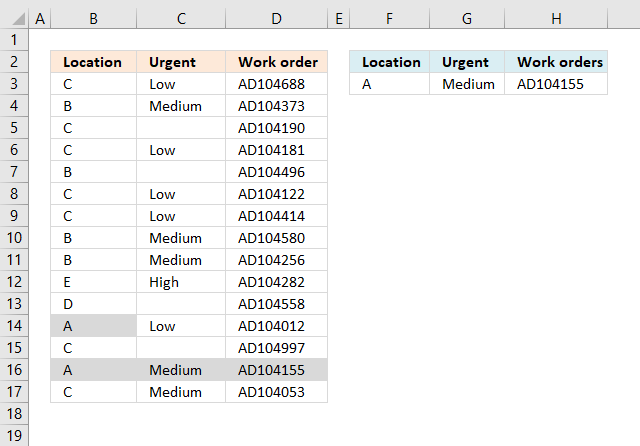The MATCH and MAX function returns the most urgent value in column C, the order of values in the array determines the importance. {0;"Low";"Medium","High"}. For example, "Medium" is more urgent than "Low". The MATCH function matches one set of values (column C) with another set of values {0;"Low";"Medium","High"}.

### Explaining the array formula in cell H3

#### Step 1 - Evaluate logical test

The IF function returns a value based on what the logical_test returns, TRUE or FALSE. This step explains how the logical_test is calculated.

IF(logical_test, [value_if_true], [value_if_false])

The equal sign allows you to compare a cell to a cell range, the parentheses lets you control the order of operation.

(\$F\$3=\$B\$3:\$B\$17)*(\$G\$3=\$C\$3:\$C\$17)

becomes

("A"={"C"; "B"; "C"; "C"; "B"; "C"; "C"; "B"; "B"; "E"; "D"; "A"; "C"; "A"; "C"})*(\$G\$3=\$C\$3:\$C\$17)

becomes

{FALSE; FALSE; FALSE; FALSE; FALSE; FALSE; FALSE; FALSE; FALSE; FALSE; FALSE; TRUE; FALSE; TRUE; FALSE}*(\$G\$3=\$C\$3:\$C\$17)

becomes

{FALSE; FALSE; FALSE; FALSE; FALSE; FALSE; FALSE; FALSE; FALSE; FALSE; FALSE; TRUE; FALSE; TRUE; FALSE}*("Medium"={"Low";"Medium";0;"Low";0;"Low";"Low";"Medium";"Medium";"High";0;"Low";0;"Medium";"Medium"})

becomes

{FALSE; FALSE; FALSE; FALSE; FALSE; FALSE; FALSE; FALSE; FALSE; FALSE; FALSE; TRUE; FALSE; TRUE; FALSE}*{FALSE; TRUE; FALSE; FALSE; FALSE; FALSE; FALSE; TRUE; TRUE; FALSE; FALSE; FALSE; FALSE; TRUE; TRUE}

and returns

{0; 0; 0; 0; 0; 0; 0; 0; 0; 0; 0; 0; 0; 1; 0}

If you multiply a boolean value with a boolean value the result is always the numerical equivalent. TRUE -> 1 and FALSE -> 0 (zero)

#### Step 2 - Return corresponding values

The IF function replaces the array containing boolean values with values based on the outcome of the logical_test, TRUE (1) returns the second argument [value_if_true] and FALSE (0) returns the third argument [value_if_false].

IF(logical_test, [value_if_true], [value_if_false])

IF((\$F\$3=\$B\$3:\$B\$17)*(\$G\$3=\$C\$3:\$C\$17), MATCH(ROW(\$B\$3:\$B\$17), ROW(\$B\$3:\$B\$17)), "")

The second argument contains these functions:

MATCH(ROW(\$B\$3:\$B\$17), ROW(\$B\$3:\$B\$17))

It creates an array containing a sequence from 1 to 15.

MATCH(ROW(\$B\$3:\$B\$17), ROW(\$B\$3:\$B\$17))

becomes

MATCH({3; 4; 5; 6; 7; 8; 9; 10; 11; 12; 13; 14; 15; 16; 17}, {3; 4; 5; 6; 7; 8; 9; 10; 11; 12; 13; 14; 15; 16; 17})

and returns

{1; 2; 3; 4; 5; 6; 7; 8; 9; 10; 11; 12; 13; 14; 15}

IF((\$F\$3=\$B\$3:\$B\$17)*(\$G\$3=\$C\$3:\$C\$17), MATCH(ROW(\$B\$3:\$B\$17), ROW(\$B\$3:\$B\$17)), "")

becomes

IF({0; 0; 0; 0; 0; 0; 0; 0; 0; 0; 0; 0; 0; 1; 0}, MATCH(ROW(\$B\$3:\$B\$17), ROW(\$B\$3:\$B\$17)), "")

becomes

IF({0; 0; 0; 0; 0; 0; 0; 0; 0; 0; 0; 0; 0; 1; 0}, {1; 2; 3; 4; 5; 6; 7; 8; 9; 10; 11; 12; 13; 14; 15}, "")

and returns

{""; ""; ""; ""; ""; ""; ""; ""; ""; ""; ""; ""; ""; 14; ""}

#### Step 3 - Extract k-th smallest number

The SMALL function returns the k-th smallest number ina cell range or array. It ignores blanks and text values, however, not error values.

SMALL(arrayk)

SMALL(IF((\$F\$3=\$B\$3:\$B\$17)*(\$G\$3=\$C\$3:\$C\$17), MATCH(ROW(\$B\$3:\$B\$17), ROW(\$B\$3:\$B\$17)), ""), ROW(A1))

becomes

SMALL({""; ""; ""; ""; ""; ""; ""; ""; ""; ""; ""; ""; ""; 14; ""}, ROW(A1))

becomes

SMALL({""; ""; ""; ""; ""; ""; ""; ""; ""; ""; ""; ""; ""; 14; ""}, 1)

and returns 14.

#### Step 4 - Return value

The INDEX function returns a value from a table based on row and column number. The column number is optional if you only fetch values from a single column.

INDEX(array[row_num][column_num])

INDEX(\$D\$3:\$D\$17, SMALL(IF((\$F\$3=\$B\$3:\$B\$17)*(\$G\$3=\$C\$3:\$C\$17), MATCH(ROW(\$B\$3:\$B\$17), ROW(\$B\$3:\$B\$17)), ""),ROW(A1)))

becomes

INDEX(\$D\$3:\$D\$17, 14)

and returns "AD104155" in cell H3.

#### Step 5 - Catch error

The IFERROR function traps errors and allows you to return any value if an error has occurred. The IFERROR function returns a blank cell if an error is returned.

Be careful with the IFERROR function, it can make it very difficult to find errors in a worksheet.

IFERROR(valuevalue_if_error)

IFERROR(INDEX(\$D\$3:\$D\$17, SMALL(IF((\$F\$3=\$B\$3:\$B\$17)*(\$G\$3=\$C\$3:\$C\$17), MATCH(ROW(\$B\$3:\$B\$17), ROW(\$B\$3:\$B\$17)), ""),ROW(A1))), "")

### Get the Excel fileFind-most-urgent-work-orders.xlsx